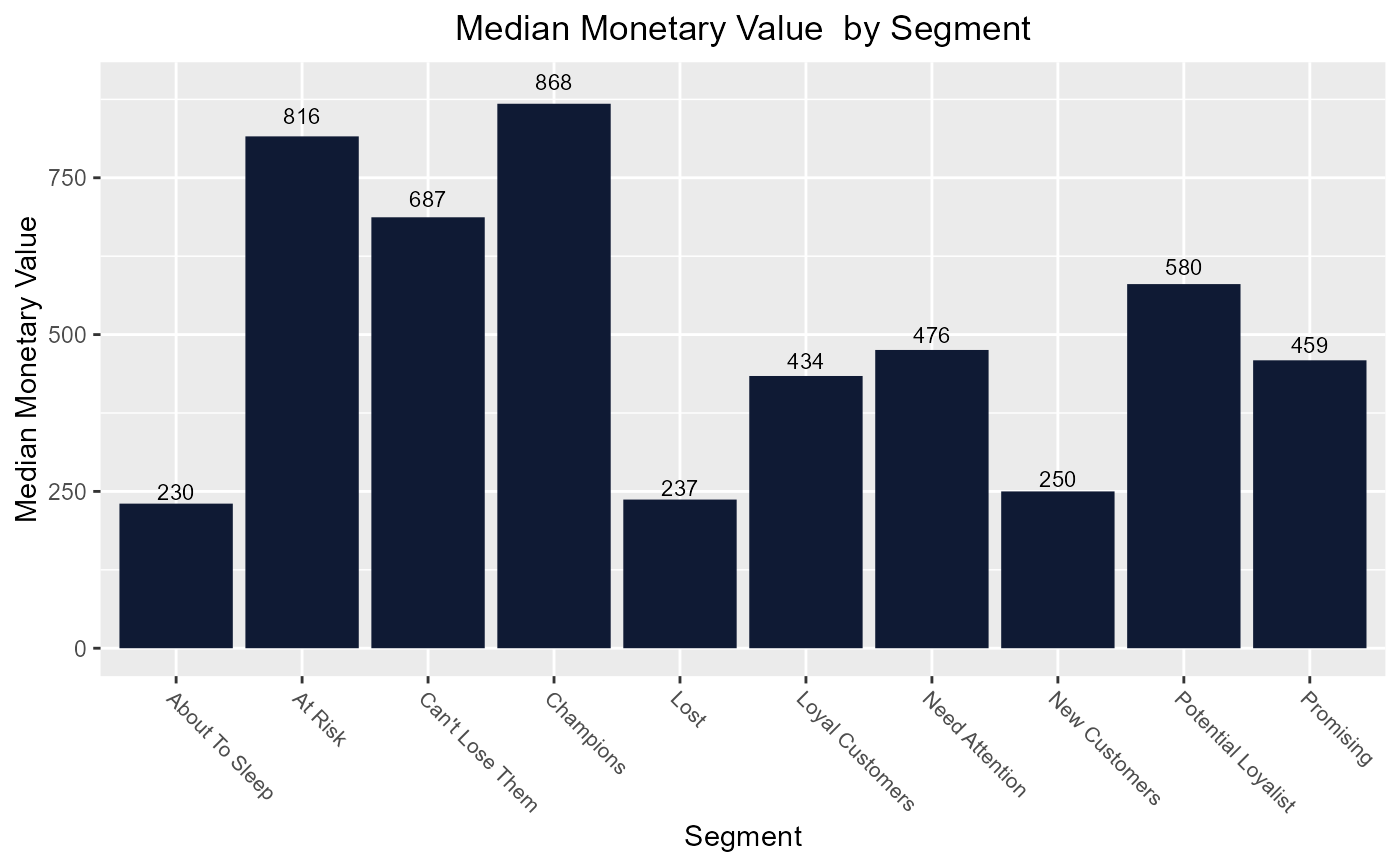Segment wise median recency, frequency & monetary value plot.

## Usage

rfm_plot_median_recency(
rfm_segment_table,
color = "blue",
font_size = 6,
sort = FALSE,
ascending = FALSE,
flip = FALSE,
print_plot = TRUE
)

rfm_plot_median_frequency(
rfm_segment_table,
color = "blue",
font_size = 6,
sort = FALSE,
ascending = FALSE,
flip = FALSE,
print_plot = TRUE
)

rfm_plot_median_monetary(
rfm_segment_table,
color = "blue",
font_size = 6,
sort = FALSE,
ascending = FALSE,
flip = FALSE,
print_plot = TRUE
)

## Arguments

rfm_segment_table

Output from rfm_segment.

color

Color of the bars.

font_size

Font size for X axis text.

sort

logical; if TRUE, sort metrics.

ascending

logical; if TRUE, sort metrics in ascending order.

flip

logical; if TRUE, creates horizontal bar plot.

print_plot

logical; if TRUE, prints the plot else returns a plot object.

## Examples

# analysis date
analysis_date <- as.Date('2006-12-31')

# generate rfm score
rfm_result <- rfm_table_order(rfm_data_orders, customer_id, order_date,
revenue, analysis_date)

# segment names
segment_names <- c("Champions", "Potential Loyalist", "Loyal Customers",
"Promising", "New Customers", "Can't Lose Them",
"At Risk", "Need Attention", "About To Sleep", "Lost")

# segment intervals
recency_lower <-   c(5, 3, 2, 3, 4, 1, 1, 1, 2, 1)
recency_upper <-   c(5, 5, 4, 4, 5, 2, 2, 3, 3, 1)
frequency_lower <- c(5, 3, 2, 1, 1, 3, 2, 3, 1, 1)
frequency_upper <- c(5, 5, 4, 3, 3, 4, 5, 5, 3, 5)
monetary_lower <-  c(5, 2, 2, 3, 1, 4, 4, 3, 1, 1)
monetary_upper <-  c(5, 5, 4, 5, 5, 5, 5, 5, 4, 5)

# generate segments
segments <- rfm_segment(rfm_result, segment_names, recency_lower,
recency_upper, frequency_lower, frequency_upper, monetary_lower,
monetary_upper)

# plots
# visualize median recency
rfm_plot_median_recency(segments)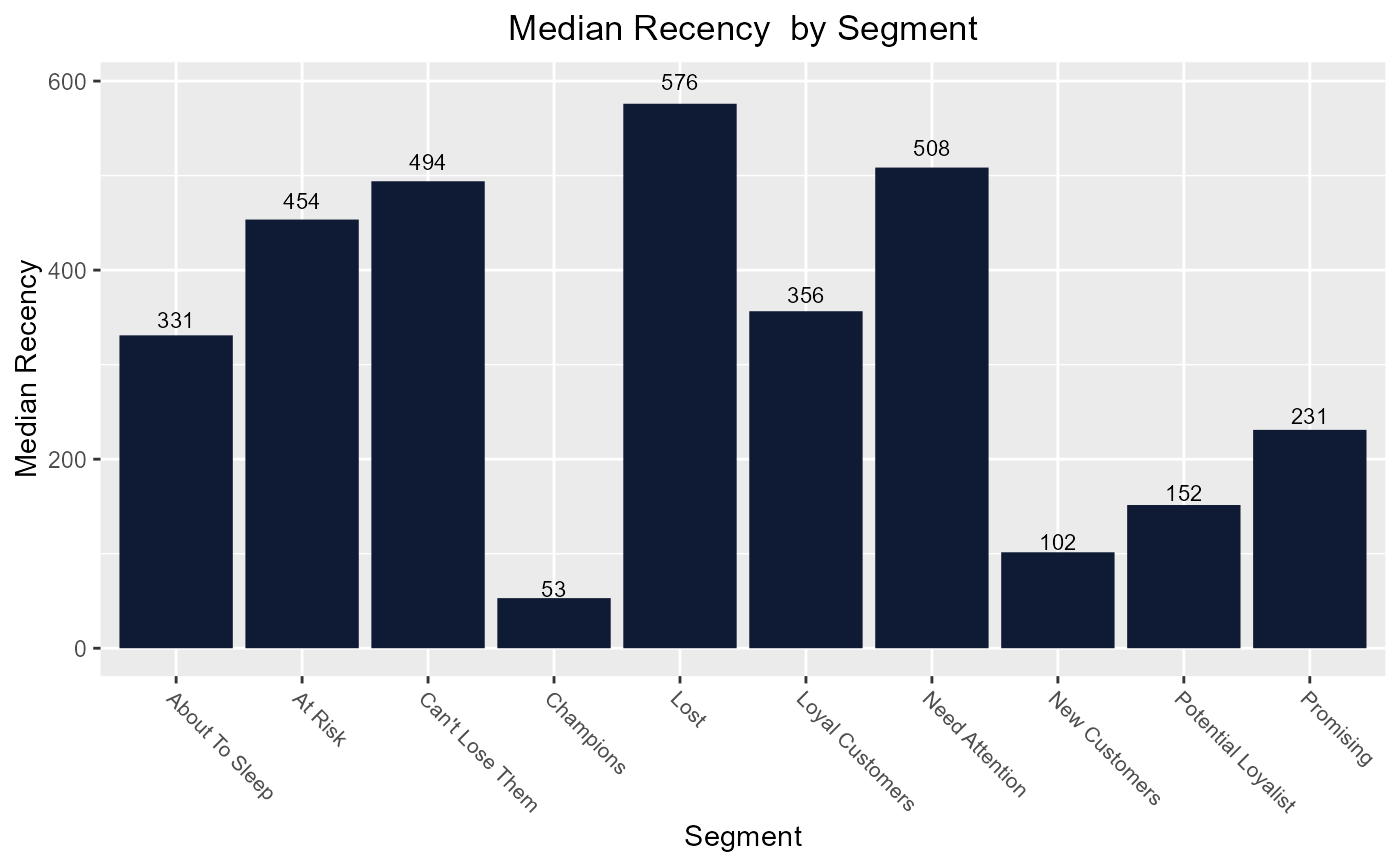# sort in ascending order
rfm_plot_median_recency(segments, sort = TRUE, ascending = TRUE)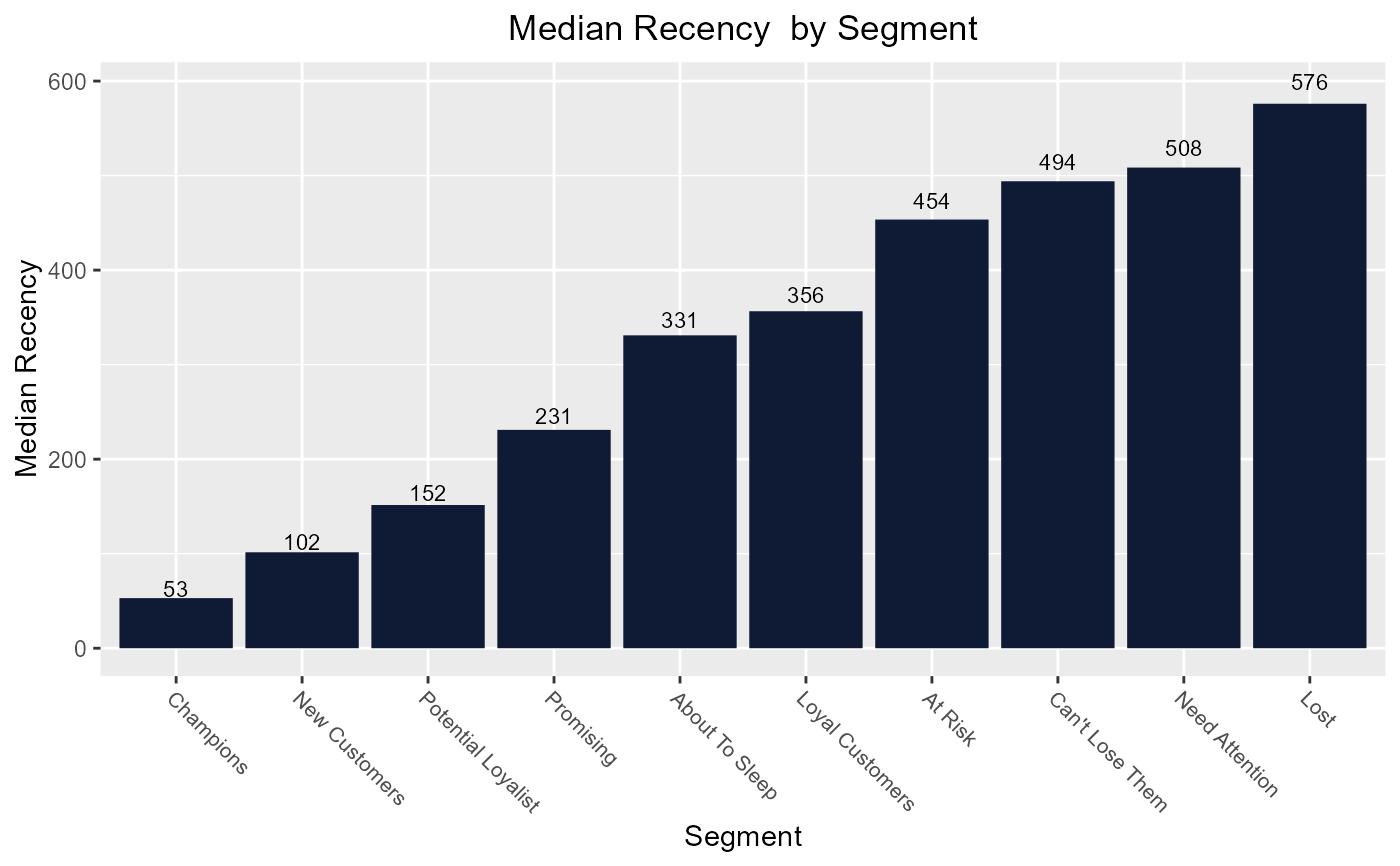# default sorting is in descending order
rfm_plot_median_recency(segments, sort = TRUE)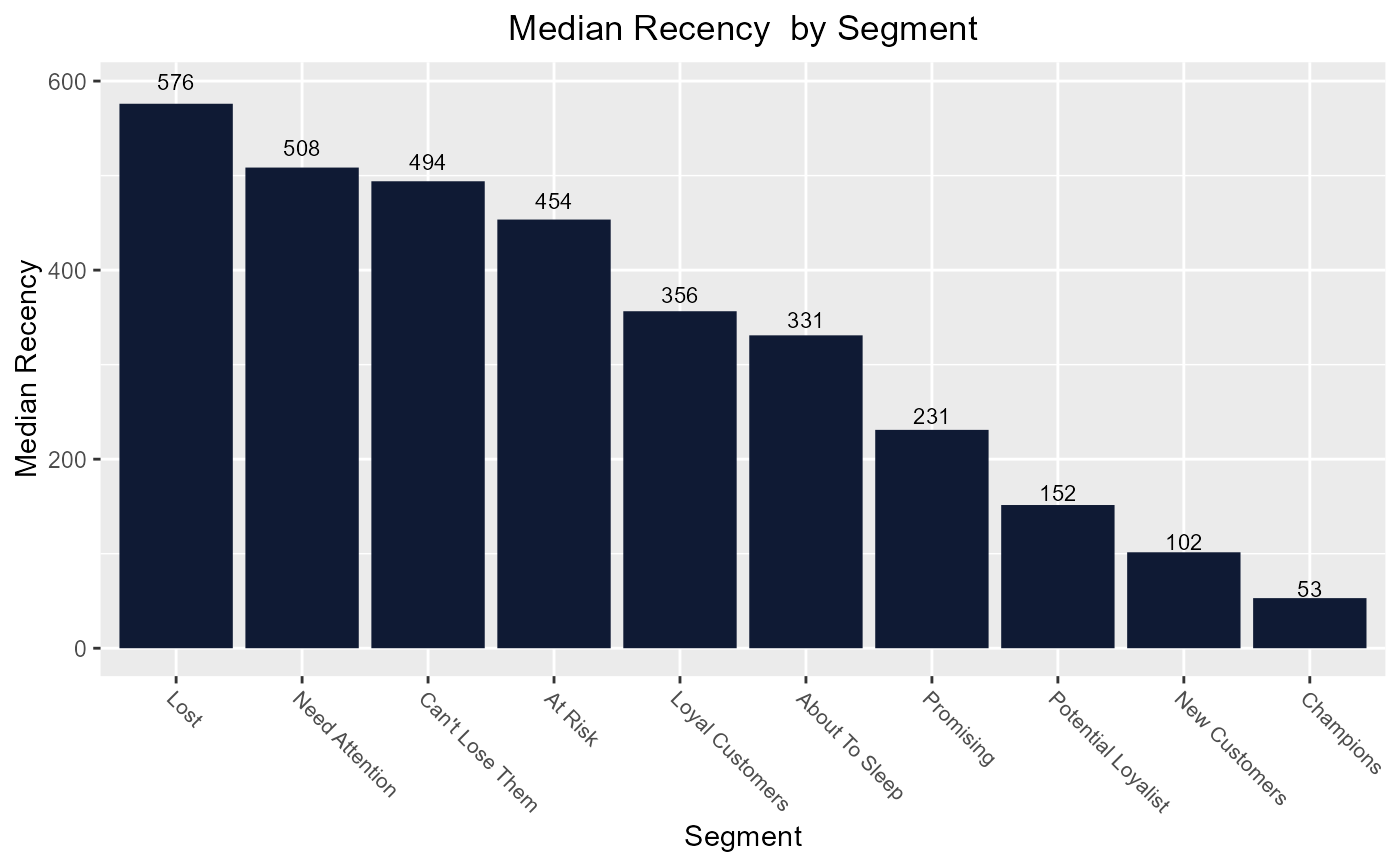# horizontal bars
rfm_plot_median_recency(segments, flip = TRUE)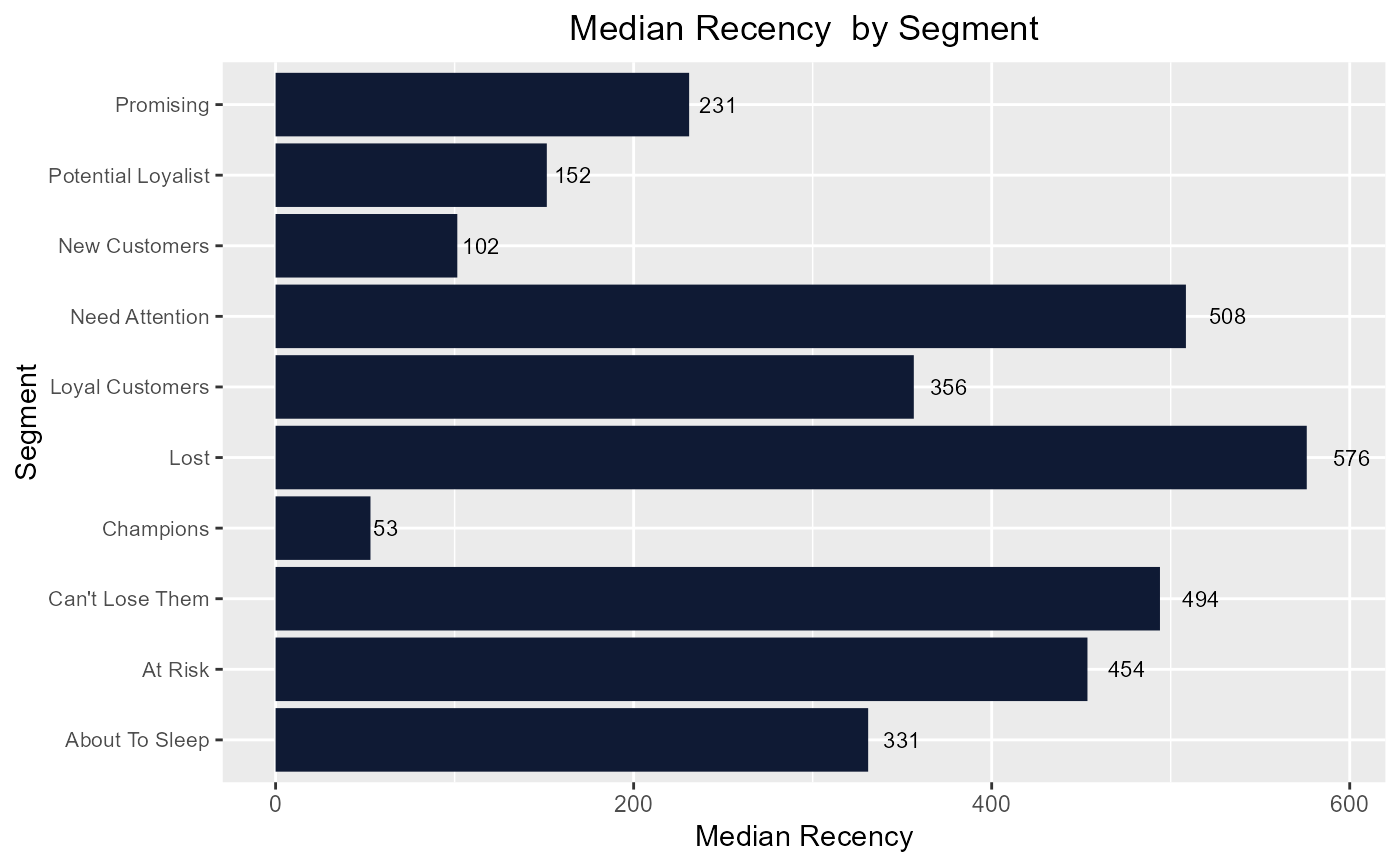# median frequency
rfm_plot_median_frequency(segments)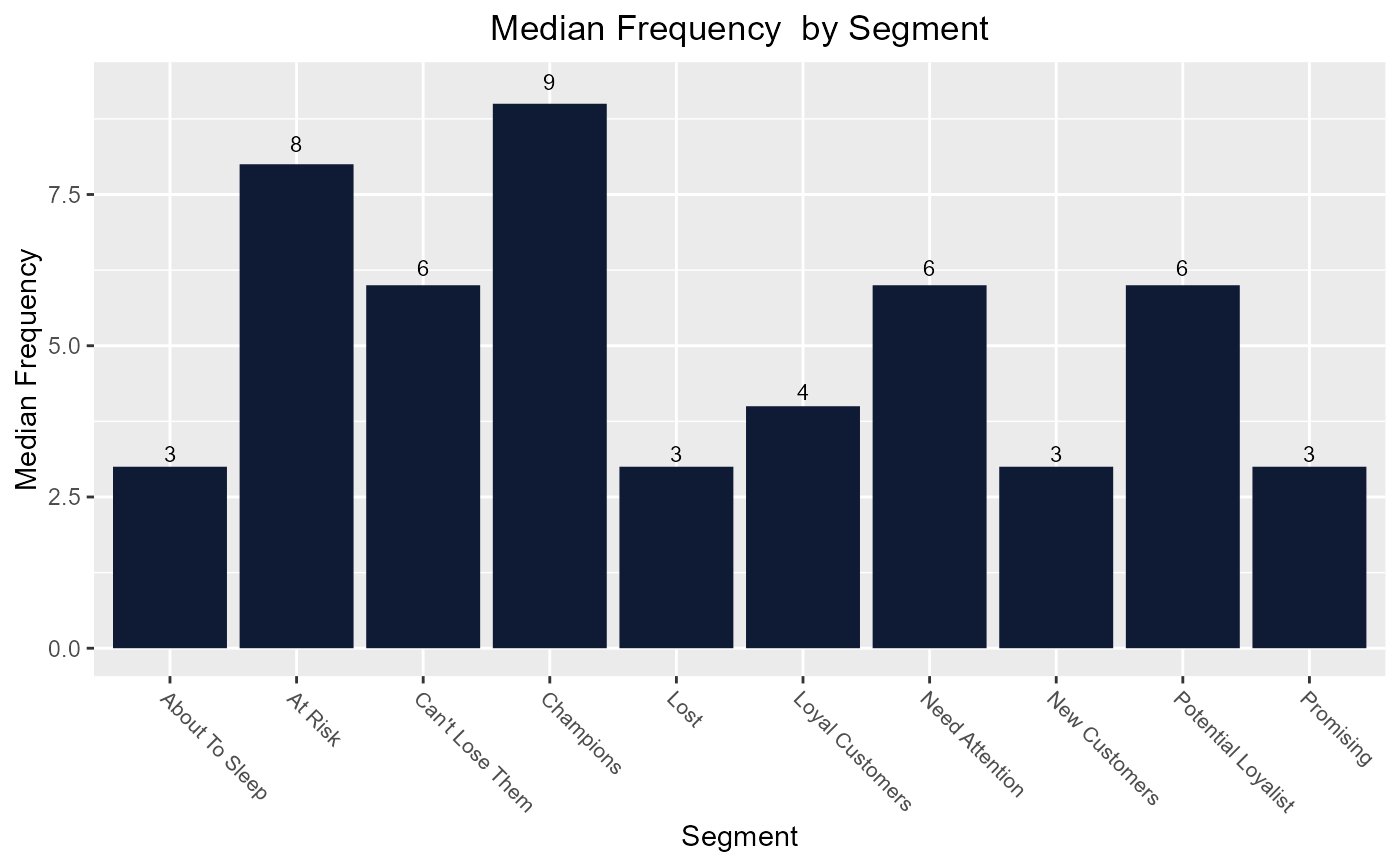# median monetary value
rfm_plot_median_monetary(segments)